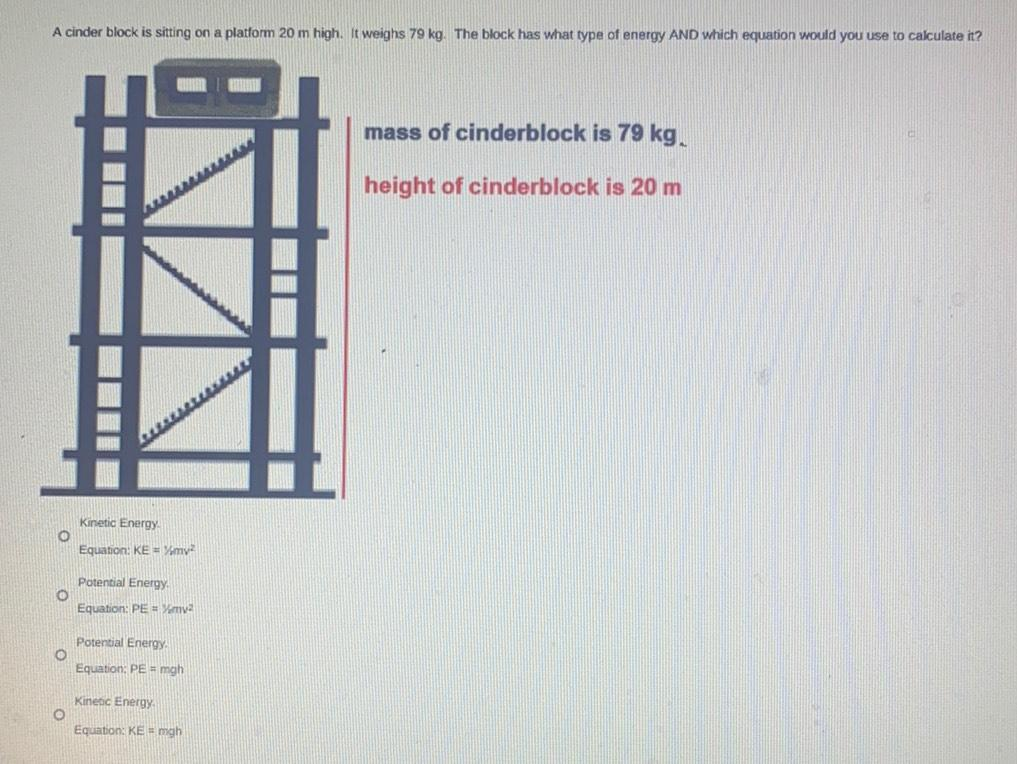Question:

# A cinder block is sitting on a platform 20 m high. It weighs 79 kg. The block has what type of energy AND which equation would you use to calculate it? (A)Kinetic Energy Equation: KE= 1/2mv² (B)PotentA cinder block is sitting on a platform 20 m high. It weighs 79 kg. The block has what type of energy AND which equation would you use to calculate it? (A)Kinetic Energy Equation: KE= 1/2mv² (B)Potential Energy Equation: PE = 1/2mv² (C)Potential Energy Equation: PE = mgh (D)Kinetic Energy Equation: KE = mgh